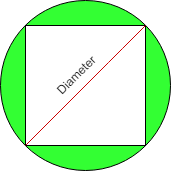# Find area of the Circle when the area of inscribed Square is given

• Last Updated : 24 Nov, 2021

Given area of square inscribed in a circle as N, the task is to calculate the area of circle in which the square is inscribed.

Examples:

Attention reader! Don’t stop learning now. Get hold of all the important DSA concepts with the DSA Self Paced Course at a student-friendly price and become industry ready.  To complete your preparation from learning a language to DS Algo and many more,  please refer Complete Interview Preparation Course.

In case you wish to attend live classes with experts, please refer DSA Live Classes for Working Professionals and Competitive Programming Live for Students.

Input: N = 4
Output: 6.283

Input: N = 10
Output:  15.707

Approach:

Consider the below image:• Let the area of the square is ‘A’
• The side of the square is given by = A**(1/2)
• A right-angled triangle is formed by the two sides of the square and the diameter of the circle
• The hypotenuse of triangle will be diameter of circle
• The diameter of circle ‘D’ is calculated as ((A * A) + (A * A))**(1/2)
• The radius of circle ‘r’ is given by D/2
• The resultant area of the circle is pi*r*r

Below is the implementation of the above approach:

## C++

 `#include ``#include``#include ``using` `namespace` `std;` `// Function to calculate the area of circle``double` `areaOfCircle(``double` `a)``{``    ``// declaring pi``    ``double` `pi=2*``acos``(0.0);``      ` `    ``// Side of the square``    ``double` `side = ``pow``(a,(1.0 / 2));` `    ``// Diameter of circle``    ``double` `D =``pow``( ((side * side) + (side * side)) ,(1.0 / 2));` `    ``// Radius of circle``    ``double` `R = D / 2;` `    ``// Area of circle``    ``double` `Area = pi * (R * R);` `    ``return` `Area;` `}`` ``//Driver Code``int` `main() {``   ` `    ``double` `areaOfSquare = 4;``    ``cout<

## Java

 `// Java code for the above approach``import` `java.util.*;` `class` `GFG``{``  ` `    ``// Function to calculate the area of circle``    ``static` `double` `areaOfCircle(``double` `a)``    ``{` `        ``// Side of the square``        ``double` `side = Math.pow(a, (``1.0` `/ ``2``));` `        ``// Diameter of circle``        ``double` `D = Math.pow(((side * side) + (side * side)),``                            ``(``1.0` `/ ``2``));` `        ``// Radius of circle``        ``double` `R = D / ``2``;` `        ``// Area of circle``        ``double` `Area = Math.PI * (R * R);` `        ``return` `Area;``    ``}``  ` `    ``// Driver Code``    ``public` `static` `void` `main(String[] args)``    ``{``        ``double` `areaOfSquare = ``4``;``        ``System.out.println(areaOfCircle(areaOfSquare));``    ``}``}` `// This code is contribute by Potta Lokesh`

## Python3

 `# Python program for the above approach``import` `math` `# Function to calculate the area of circle``def` `areaOfCircle(a):` `    ``# Side of the square``    ``side ``=` `a``*``*``(``1` `/` `2``)` `    ``# Diameter of circle``    ``D ``=` `((side ``*` `side) ``+` `(side ``*` `side))``*``*``(``1` `/` `2``)` `    ``# Radius of circle``    ``R ``=` `D ``/` `2` `    ``# Area of circle``    ``Area ``=` `math.pi ``*` `(R ``*` `R)` `    ``return` `Area` `# Driver Code``areaOfSquare ``=` `4``print``(areaOfCircle(areaOfSquare))`

## C#

 `// C# code for the above approach``using` `System;` `class` `GFG``{``  ` `    ``// Function to calculate the area of circle``    ``static` `double` `areaOfCircle(``double` `a)``    ``{` `        ``// Side of the square``        ``double` `side = Math.Pow(a, (1.0 / 2));` `        ``// Diameter of circle``        ``double` `D = Math.Pow(((side * side) + (side * side)),``                            ``(1.0 / 2));` `        ``// Radius of circle``        ``double` `R = D / 2;` `        ``// Area of circle``        ``double` `Area = Math.PI * (R * R);` `        ``return` `Area;``    ``}``  ` `    ``// Driver Code``    ``public` `static` `void` `Main()``    ``{``        ``double` `areaOfSquare = 4;``        ``Console.Write(areaOfCircle(areaOfSquare));``    ``}``}` `// This code is contribute by Samim Hossain Mondal.`

## Javascript

 ``
Output
`6.283185307179588`

Time Complexity: O(1)
Auxiliary Space: O(1)

My Personal Notes arrow_drop_up